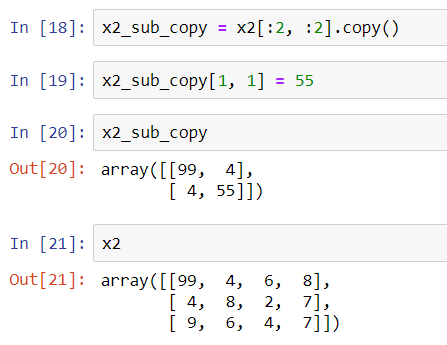Prerequisite: Array Indexing in NumPy

Slicing an array in NumPy means to extract a subarray out of the complete array. We can then perform operations on the subarray only.

Just as we use square brackets to extract individual array elements, we can use the same to extract subarrays, using this notation - x[start: stop: step]

If any of these is not mentioned, the default values are used. The default values of each of these are: start = 0, stop = size of the array, step = 1.

### One-Dimensional Subarrays

Let us begin by creating a one-dimensional NumPy array.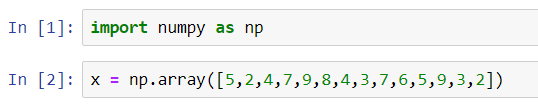#### Subarray of first n elements

In the below given example, we print a subarray of the first 5 elements.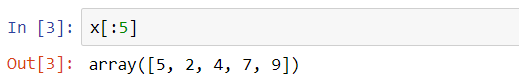#### Elements after index n

In the below given example, we print a subarray of elements after index 5.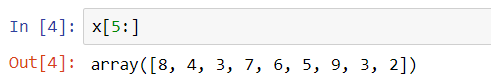#### Middle subarray

In the below given example, we print a subarray of elements starting from index 3 and ending with index 9.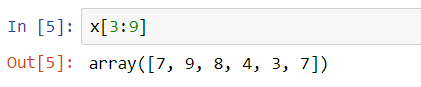#### Every kth element

In the below given example, we print a subarray of every 2nd element.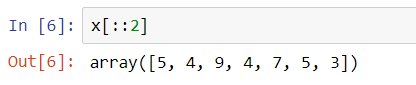#### Every kth element, starting from index i

In the below given example, we print a subarray of every 4th element starting from index 2.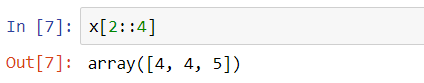#### Slicing subarrays in reverse order

In the below given example, we print a subarray of elements in reverse order between indices 2 and 5.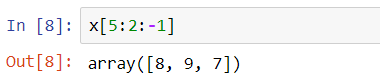### Multidimensional Subarrays

We can perform slicing in multidimensional arrays too. Let us begin by first creating a 2D array.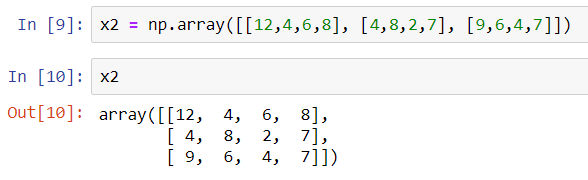#### Subarray of n rows and m columns

In the below given example, we print a subarray of 2 rows and 3 columns.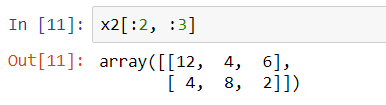#### All rows every mth column

In the below given example, we print a subarray of all rows and every 2nd column.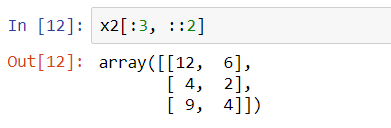#### All rows and columns in reverse order

In the below given example, we print a subarray of the rows and columns, but in reverse order.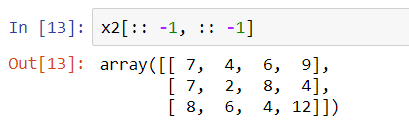### Subarrays as no-copy views

We shall see the difference between NumPy arrays and Python lists here.

When we slice a NumPy array, it returns a view rather than a copy of the data. In Python lists, slices will be copies.

Let us understand this through an example.

We have sliced a subarray of 2 rows and 2 columns and stored it in x2_sub. We try to give a new value at index (0,0) in the subarray, not in the original array. But we can see, that when we reassign a new value in the subarray, changes are reflected in the original array too.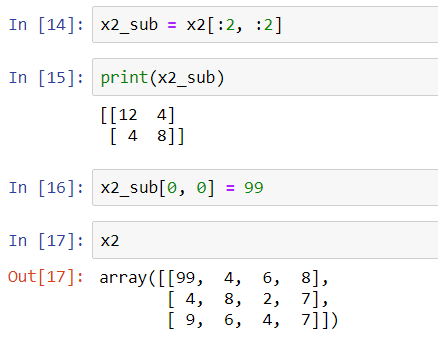### Creating copies of arrays

One way to create copies of subarrays after slicing is to use a copy function.

In the below example we can see that changing any value in the subarray does not bring any change in the original array.